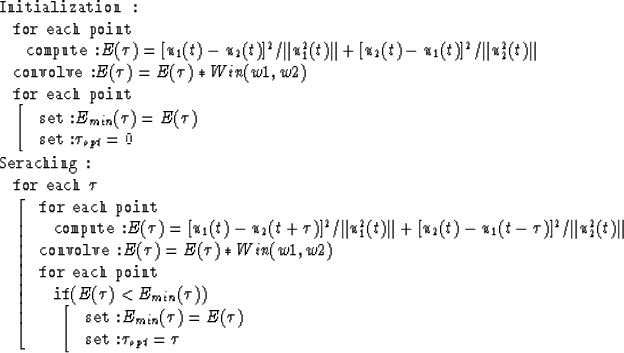Next: LINEAR OPTIMIZATION Up: NON-LINEAR OPTIMIZATION Previous: Objective function

## Algorithm

Up till now, we have treatedas a constant. In the real world, however, the seismic reflections are almost always curved events. Therefore,changes its value from place to place. To deal with this nonstationarity, we consider the curved events as piecewise linear and chop a seismic section into many subsections. The optimalin a subsection is the minimizer of the objective function that is computed from the data samples in the subsection. This is a one-dimensional non-linear optimization problem and can be conveniently solved by searching. A straight forward implementation of the algorithm is to read the data samples in a subsection, then to compute the objective function for all possible, and finally to find thethat minimizes the objection function. These steps are repeated for every subsection. This implementation is actually cumbersome and computationally expensive because many boundaries must be properly handled and the objective function may be computed more than once at the points shared by several subsections.

We have found an efficient way to implement the algorithm. The scheme of the implementation is:The only kind of boundaries that the program has to deal with is the truncation of data, but it can be easily handled by padding zeros. The code of this implementation vectorizes fully.Next: LINEAR OPTIMIZATION Up: NON-LINEAR OPTIMIZATION Previous: Objective function
Stanford Exploration Project
1/13/1998